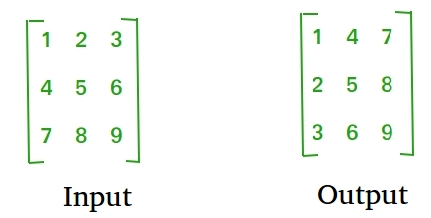Java Program to find transpose of a matrix

• Last Updated : 07 Nov, 2018

Transpose of a matrix is obtained by changing rows to columns and columns to rows. In other words, transpose of A[][] is obtained by changing A[i][j] to A[j][i].For Square Matrix :

The below program finds transpose of A[][] and stores the result in B[][], we can change N for different dimension.

 // Java Program to find // transpose of a matrix  class GFG{    static final int N = 4;          // This function stores transpose    // of A[][] in B[][]    static void transpose(int A[][], int B[][])    {        int i, j;        for (i = 0; i < N; i++)            for (j = 0; j < N; j++)                B[i][j] = A[j][i];    }          // Driver code    public static void main (String[] args)    {        int A[][] = { {1, 1, 1, 1},                      {2, 2, 2, 2},                      {3, 3, 3, 3},                      {4, 4, 4, 4}};              int B[][] = new int[N][N], i, j;              transpose(A, B);              System.out.print("Result matrix is \n");        for (i = 0; i < N; i++)        {            for (j = 0; j < N; j++)            System.out.print(B[i][j] + " ");            System.out.print("\n");        }    }}  // This code is contributed by Anant Agarwal.
Output:
Result matrix is
1 2 3 4
1 2 3 4
1 2 3 4
1 2 3 4

For Rectangular Matrix :

The below program finds transpose of A[][] and stores the result in B[][].

 // Java Program to find // transpose of a matrix  class GFG{    static final int M = 3;    static final int N = 4;          // This function stores transpose    // of A[][] in B[][]    static void transpose(int A[][], int B[][])    {        int i, j;        for (i = 0; i < N; i++)            for (j = 0; j < M; j++)                B[i][j] = A[j][i];    }          // Driver code    public static void main (String[] args)    {        int A[][] = { {1, 1, 1, 1},                      {2, 2, 2, 2},                      {3, 3, 3, 3}};              int B[][] = new int[N][M], i, j;              transpose(A, B);              System.out.print("Result matrix is \n");        for (i = 0; i < N; i++)        {            for (j = 0; j < M; j++)            System.out.print(B[i][j] + " ");            System.out.print("\n");        }    }}  // This code is contributed by Anant Agarwal.
Output:
Result matrix is
1 2 3
1 2 3
1 2 3
1 2 3

In-Place for Square Matrix:

 // Java Program to find // transpose of a matrix  class GFG{    static final int N = 4;          // Finds transpose of A[][] in-place    static void transpose(int A[][])    {        for (int i = 0; i < N; i++)            for (int j = i+1; j < N; j++)            {                 int temp = A[i][j];                 A[i][j] = A[j][i];                 A[j][i] = temp;            }    }          // Driver code    public static void main (String[] args)    {        int A[][] = { {1, 1, 1, 1},                      {2, 2, 2, 2},                      {3, 3, 3, 3},                      {4, 4, 4, 4}};                 transpose(A);              System.out.print("Modified matrix is \n");        for (int i = 0; i < N; i++)        {            for (int j = 0; j < N; j++)            System.out.print(A[i][j] + " ");            System.out.print("\n");        }    }}
Output:
Modified matrix is
1 2 3 4
1 2 3 4
1 2 3 4
1 2 3 4

Please refer complete article on Program to find transpose of a matrix for more details!

My Personal Notes arrow_drop_up